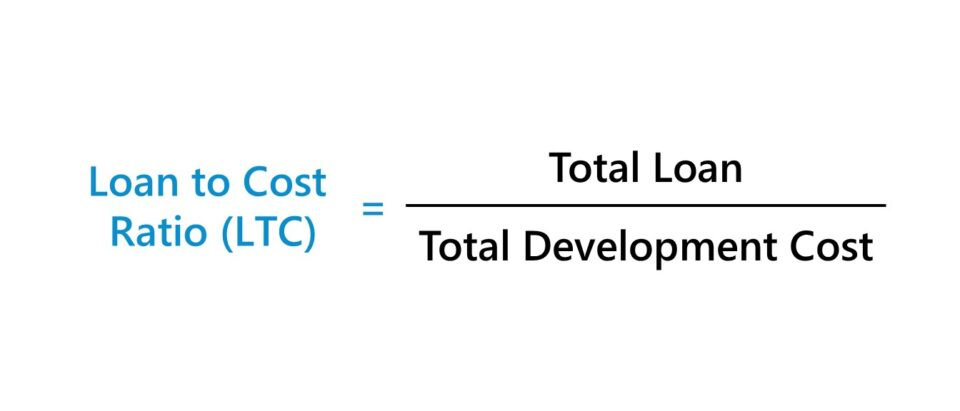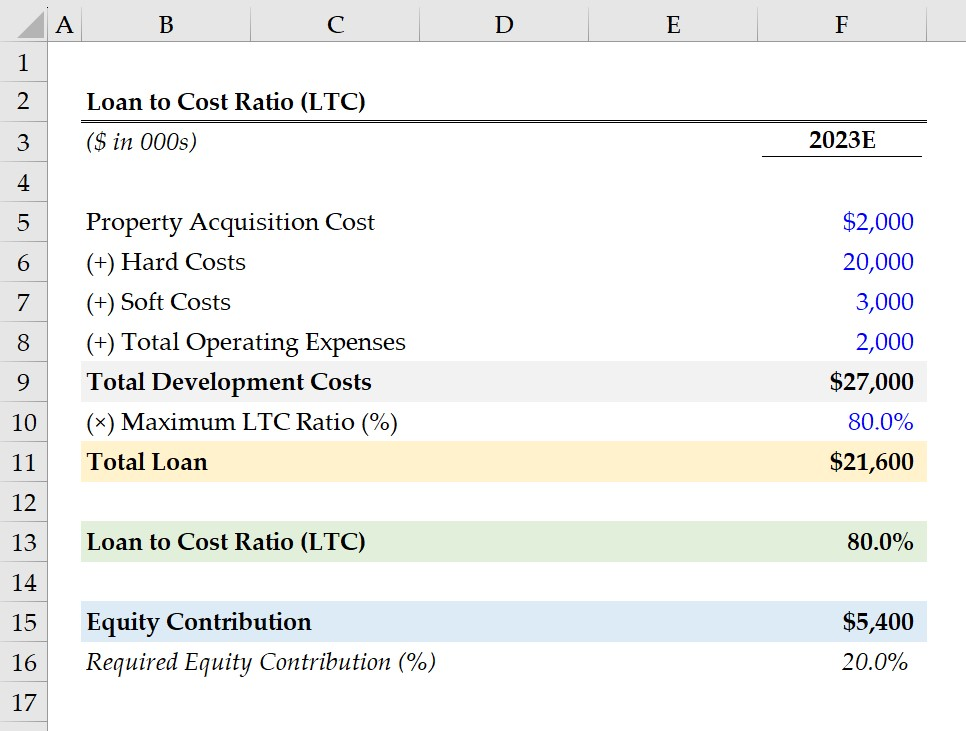Welcome to Wall Street Prep! Use code at checkout for 15% off.# Loan to Cost Ratio (LTC)

Guide to Understanding the Loan to Cost Ratio (LTC)## How to Calculate Loan to Cost Ratio (LTC)?

From the perspective of lenders underwriting a loan, the loan to cost (LTC) measures the riskiness of a requested loan by comparing the total loan amount to the total cost of the project.

• Total Loan → The total size of the loan provided by the lender to financing the real estate project.
• Total Project Cost → The cost component depends on the context of the project. For instance, the cost in a development project is likely to be the construction costs, or the purchase price in acquisition projects.

The loan to cost (LTC) is often used in conjunction with the loan to value ratio (LTV) and the debt service coverage (DSCR) ratio to determine the risk profile of a potential borrower.

The real estate lender’s decision on the loan request, the sizing of the loan, and the financing terms, such as interest rate pricing, are predicated on the borrower’s credit risk.

Each ratio is a measure of risk to gauge the likelihood of the borrower defaulting on the loan.

But unique to the loan to cost ratio (LTC), the metric is perceived as more conservative, which appeals to real estate lenders that prioritize capital preservation over yield.

The LTC ratio is a tool for lenders to gauge the risk posed by a requested loan to fund a project, whereas real estate investors use the ratio to determine their required equity contribution to complete the project.## Loan to Cost Ratio Formula (LTC)

The loan to cost ratio (LTC) formula divides the total loan amount by the total development cost of the project.

Loan to Cost Ratio (LTC) = Total Loan ÷ Total Development Cost

Since the LTC ratio is expressed as a percentage, the resulting figure must then be multiplied by 100.

The “Total Development Cost” includes the following cost categories:

• Hard Costs → Construction Materials and Labor Costs, Site Work Activities, Utilities Set-Up (HVAC), Landscaping, Parking Lot and Paving Costs
• Soft Costs → Architectural Design, Engineering Planning, Inspection and Permit Fees, Professional Services (Legal, Accounting Fees), Maintenance and Insurance Costs
• Property Acquisition Costs → Land Acquisition, Property Purchase Price
• Operating Expenses → General and Administrative (G&A), Security Fees, Property Management Fees, Payroll, Marketing, Advertising

In comparison, the loan to value ratio (LTV) divides the total loan amount by the property’s fair value. The property value is based on an appraisal of its current fair market value (FMV).

Loan to Value Ratio (LTV) = Total Loan ÷ Property Value

## What is a Good Loan to Cost Ratio?

The maximum loan to cost ratio (LTC) requirement is specific to a given lender and can be affected by external factors, such as the current interest rate environment, the supply/demand conditions in the real estate market, and economic outlook.

Generally, the maximum loan to cost ratio (LTC) requirement set by lenders in the commercial real estate market (CRE) is between 80% to 90%.

Therefore, borrowers must fulfill a 10% to 20% down payment – i.e. equity contribution – as part of the financing agreement.

Most institutional real estate lenders also set a constraint on the maximum LTV ratio, which tends to be around 70% to 80%. The lower LTV ratio requirement range reaffirms the notion that lenders in pursuit of higher yields prefer to utilize the LTV ratio in their underwriting analysis more than risk-averse, conservative lenders.

A higher loan to cost ratio (LTC) implies greater risk because the borrower has contributed less equity in the total financing of the project (and vice versa for lower ratios).

The lower the borrower’s equity contribution (i.e. less “skin in the game”), the higher the likelihood that the lender will not approve the financing because of the disproportionate allocation of risk placed on the lender.

## Loan to Cost (LTC) vs. Loan to Value Ratio (LTV): What is the Difference?

In the real estate underwriting process, the loan to cost (LTC) and loan to value (LTV) are complementary financial ratios that each provide practical insights around debt sizing.

• Loan to Cost Ratio (LTC) → The loan to cost ratio (LTC) is more often used in the context of real estate development properties and similar projects with substantial up-front costs on the initial purchase date. If the financing arrangement relies more on the LTC ratio, the lender is willing to provide the loan as a percentage of the real estate property’s total purchase price, including renovation and repair costs.
• Loan to Value Ratio (LTV) → The loan to value ratio (LTV) is the ratio between the total loan amount and the fair market value (FMV) of the project, i.e. the underlying real estate asset, after completion. The usage of the LTV ratio tends to be more common for existing rental properties and stabilized real estate projects, i.e. the property investment is functional with renovations, repairs and other improvements are complete. If the financing arrangement relies more on the LTV ratio rather than the LTC ratio, then the lender is willing to provide the loan as a percentage of the underlying real estate property’s fair value.

With that said, real estate financing arrangements where the loan to cost ratio (LTC) is the main determinant of the loan size and terms are perceived to be more lenient (and favorable) to the borrower.

However, the LTC ratio is also viewed as riskier transactions to the lender because of the increased reliance on leverage.

• Development Projects (Construction): For construction projects or real estate property investments with substantial rehabilitation or development work, the LTC ratio is better suited under such circumstances since the total development costs, such as construction costs, are considered.
• Real Estate Acquisitions (Stabilization): On the other hand, the LTV ratio is viewed as a more reliable measure of risk for real estate acquisitions because the fair market value (FMV) of the property is improbable to deviate substantially from original purchase price. Of course, there are exceptions where that rule does not apply.

The decision to use the loan to cost ratio (LTC) or loan to value ratio (LTV) in the underwriting process is not mutually exclusive. But rather, the two ratios can each be used to set parameters on the loan sizing based on the estimated risk tied to the project.

## Loan to Cost Ratio Calculator (LTC)

We’ll now move on to a modeling exercise, which you can access by filling out the form below.#### Get the Excel Template!Submitting...

## 1. Commercial Real Estate Property Assumptions (CRE)

Suppose a commercial mortgage lender is performing diligence as part of the underwriting process on a real estate developer’s request for a loan to fund a long-term construction project.

The total property acquisition cost – inclusive of the land purchase price and transaction closing fees – is estimated to be \$2 million.

• Property Acquisition Price = \$2 million

The other components of the development cost are as follows.

The sum of the four costs amounts to \$27 million, which represents the total development costs of the construction project.

• Total Development Costs = \$2 million + \$20 million + \$3 million + \$2 million = \$27 million

Since we’ve determined the total capital required (\$27 million), the next step is to make an assumption for our lender’s maximum loan to cost ratio (LTC), which we’ll set at 80%.

• Maximum LTC Ratio (%) = 80.0%

After multiplying the total development costs by the maximum LTC ratio, the maximum loan amount is implied to be \$21.6 million.

Note: The total development costs neglect financing costs, such as the upfront loan origination fee, so our total development cost figure is on an unlevered basis.

## 2. Loan to Cost Ratio Calculation Example (LTC)

In the next section, we’ll start by confirming the loan to cost ratio (%) is in fact 80%.

Upon inputting our total loan and total development costs into the loan to cost ratio formula, we arrive at an LTC ratio of 80%.

• Loan to Cost Ratio (LTC) = \$21.6 million ÷ \$27 million = 80.0%

The \$21.6 million is the maximum loan size, at which the lender can maintain an LTC ratio below 80%.

From there, we’ll subtract our loan to cost ratio (LTC) of 80% from one to determine the required equity contribution in percentage form.

• Required Equity Contribution (%) = 1 – 80% = 20%

The 20% equity interest is then multiplied by the total development costs to quantify the equity contribution from the real estate developer in dollar terms, which comes out to \$5.4 million.

• Equity Contribution = 20% × \$27 million = \$5.4 million
• Total Sources of Capital = \$21.6 million (Debt) + \$5.4 million (Equity) = \$27 million20+ Hours of Online Video Training

### Master Real Estate Financial Modeling

This program breaks down everything you need to build and interpret real estate finance models. Used at the world's leading real estate private equity firms and academic institutions.

Inline Feedbacks